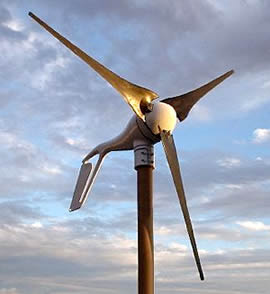A# small wind turbine annual energy outputAn estimate of the annual energy output from a wind turbine (in kilowatt-hours per year) is the best way to determine whether it and the tower will produce enough electricity to meet your needs.

A wind turbine manufacturer can help you estimate the energy production you can expect. They will use a calculation based on these factors:

• Particular wind turbine power curve
• Average annual wind speed at your site
• Height of the tower that you plan to use
• Frequency distribution of the wind – an estimate of the number of hours that the wind will blow at each speed during an average year.
•

The manufacturer should also adjust this calculation for the elevation of your site. To get a preliminary estimate of the performance of a particular wind turbine, you can use the following formula:

AEO = 0.01328 D V

Where:

• AEO = Annual energy output (kilowatt-hours [kWh]/year)
• D = Rotor diameter, feet
• V = Annual average wind speed, miles-per hour (mph), at your site
•

Note: the difference between power and energy is that power (kilowatts [kW]) is the rate at which electricity is consumed, while energy (kilowatt-hours [kWh]) is the quantity consumed.